# Usage Guide¶

This tutorial covers some basic usage patterns and best practices to help you get started with Matplotlib.

```import matplotlib.pyplot as plt
import numpy as np
```

## A simple example¶

Matplotlib graphs your data on `Figure`s (e.g., windows, Jupyter widgets, etc.), each of which can contain one or more `Axes`, an area where points can be specified in terms of x-y coordinates, or theta-r in a polar plot, x-y-z in a 3D plot, etc. The simplest way of creating a figure with an axes is using `pyplot.subplots`. We can then use `Axes.plot` to draw some data on the axes:

```fig, ax = plt.subplots()  # Create a figure containing a single axes.
ax.plot([1, 2, 3, 4], [1, 4, 2, 3])  # Plot some data on the axes.
```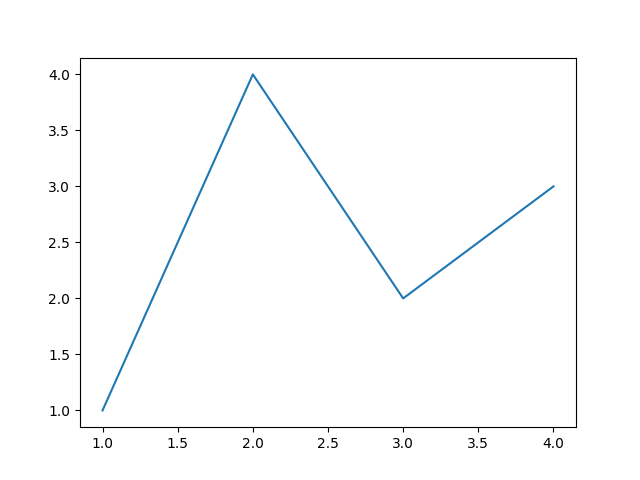Out:

```[<matplotlib.lines.Line2D object at 0x7fd1df0a02b0>]
```

Many other plotting libraries or languages do not require you to explicitly create an axes. For example, in MATLAB, one can just do

```plot([1, 2, 3, 4], [1, 4, 2, 3])  % MATLAB plot.
```

and get the desired graph.

In fact, you can do the same in Matplotlib: for each `Axes` graphing method, there is a corresponding function in the `matplotlib.pyplot` module that performs that plot on the "current" axes, creating that axes (and its parent figure) if they don't exist yet. So, the previous example can be written more shortly as

```plt.plot([1, 2, 3, 4], [1, 4, 2, 3])  # Matplotlib plot.
```Out:

```[<matplotlib.lines.Line2D object at 0x7fd1e5b59c40>]
```

## Parts of a Figure¶

Here is a more detailed layout of the components of a Matplotlib figure.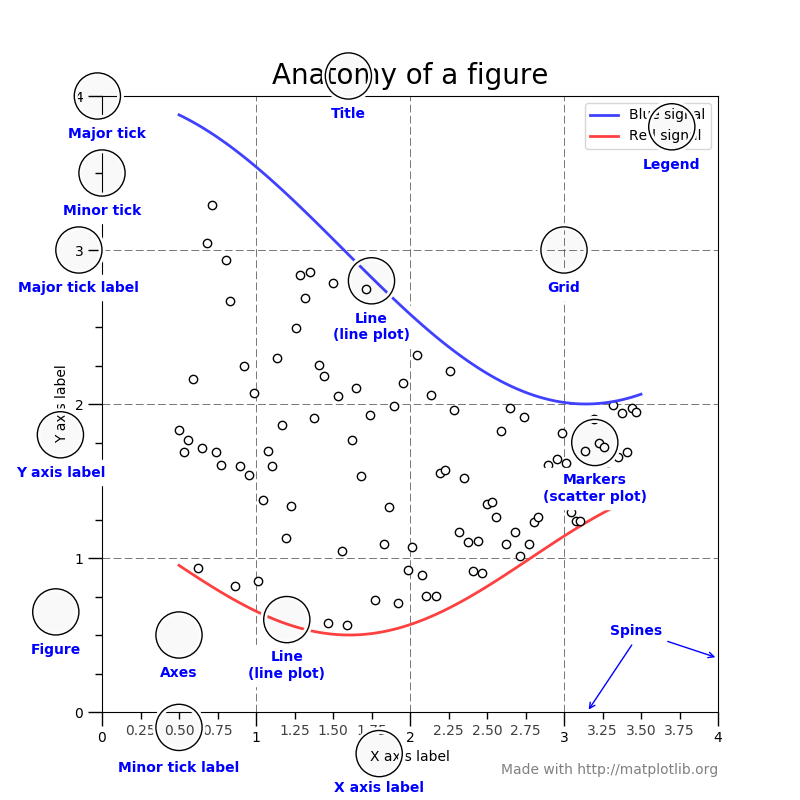### `Figure`¶

The whole figure. The figure keeps track of all the child `Axes`, a group of 'special' artists (titles, figure legends, etc), and the canvas. (The canvas is not the primary focus. It is crucial as it is the object that actually does the drawing to get you your plot, but as the user, it is mostly invisible to you). A figure can contain any number of `Axes`, but will typically have at least one.

The easiest way to create a new figure is with pyplot:

```fig = plt.figure()  # an empty figure with no Axes
fig, ax = plt.subplots()  # a figure with a single Axes
fig, axs = plt.subplots(2, 2)  # a figure with a 2x2 grid of Axes
```

It's convenient to create the axes together with the figure, but you can also add axes later on, allowing for more complex axes layouts.

### `Axes`¶

This is what you think of as 'a plot'. It is the region of the image with the data space. A given figure can contain many Axes, but a given `Axes` object can only be in one `Figure`. The Axes contains two (or three in the case of 3D) `Axis` objects (be aware of the difference between Axes and Axis) which take care of the data limits (the data limits can also be controlled via the `axes.Axes.set_xlim()` and `axes.Axes.set_ylim()` methods). Each `Axes` has a title (set via `set_title()`), an x-label (set via `set_xlabel()`), and a y-label set via `set_ylabel()`).

The `Axes` class and its member functions are the primary entry point to working with the OO interface.

### `Axis`¶

These are the objects most similar to a number line. They set graph limits and generate ticks (the marks on the axis) and ticklabels (strings labeling the ticks). The location of the ticks is determined by a `Locator` object and the ticklabel strings are formatted by a `Formatter`. The combination of the correct `Locator` and `Formatter` gives very fine control over the tick locations and labels.

### `Artist`¶

Basically, everything visible on the figure is an artist (even `Figure`, `Axes`, and `Axis` objects). This includes `Text` objects, `Line2D` objects, `collections` objects, `Patch` objects, etc... When the figure is rendered, all of the artists are drawn to the canvas. Most Artists are tied to an Axes; such an Artist cannot be shared by multiple Axes, or moved from one to another.

## Types of inputs to plotting functions¶

All of plotting functions expect `numpy.array` or `numpy.ma.masked_array` as input. Classes that are similar to arrays ('array-like') such as `pandas` data objects and `numpy.matrix` may not work as intended. Common convention is to convert these to `numpy.array` objects prior to plotting.

For example, to convert a `pandas.DataFrame`

```a = pandas.DataFrame(np.random.rand(4, 5), columns = list('abcde'))
a_asarray = a.values
```

and to convert a `numpy.matrix`

```b = np.matrix([[1, 2], [3, 4]])
b_asarray = np.asarray(b)
```

## The object-oriented interface and the pyplot interface¶

As noted above, there are essentially two ways to use Matplotlib:

• Explicitly create figures and axes, and call methods on them (the "object-oriented (OO) style").

• Rely on pyplot to automatically create and manage the figures and axes, and use pyplot functions for plotting.

So one can do (OO-style)

```x = np.linspace(0, 2, 100)  # Sample data.

# Note that even in the OO-style, we use `.pyplot.figure` to create the figure.
fig, ax = plt.subplots()  # Create a figure and an axes.
ax.plot(x, x, label='linear')  # Plot some data on the axes.
ax.plot(x, x**2, label='quadratic')  # Plot more data on the axes...
ax.plot(x, x**3, label='cubic')  # ... and some more.
ax.set_xlabel('x label')  # Add an x-label to the axes.
ax.set_ylabel('y label')  # Add a y-label to the axes.
ax.set_title("Simple Plot")  # Add a title to the axes.
```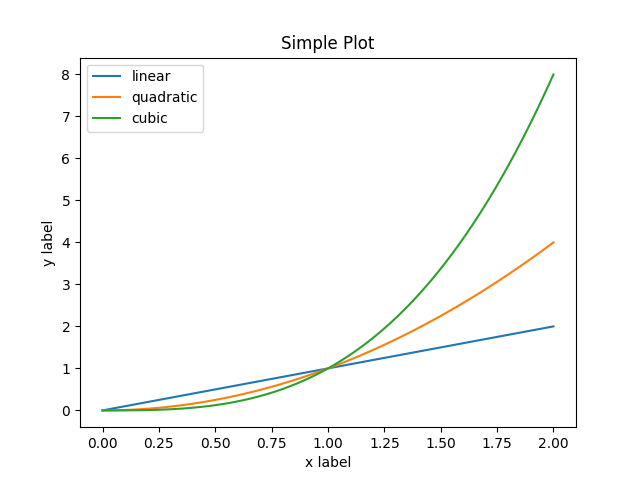Out:

```<matplotlib.legend.Legend object at 0x7fd1ded19280>
```

or (pyplot-style)

```x = np.linspace(0, 2, 100)  # Sample data.

plt.plot(x, x, label='linear')  # Plot some data on the (implicit) axes.
plt.plot(x, x**3, label='cubic')
plt.xlabel('x label')
plt.ylabel('y label')
plt.title("Simple Plot")
plt.legend()
```Out:

```<matplotlib.legend.Legend object at 0x7fd1e5dfc2e0>
```

In addition, there is a third approach, for the case when embedding Matplotlib in a GUI application, which completely drops pyplot, even for figure creation. We won't discuss it here; see the corresponding section in the gallery for more info (Embedding Matplotlib in graphical user interfaces).

Matplotlib's documentation and examples use both the OO and the pyplot approaches (which are equally powerful), and you should feel free to use either (however, it is preferable pick one of them and stick to it, instead of mixing them). In general, we suggest to restrict pyplot to interactive plotting (e.g., in a Jupyter notebook), and to prefer the OO-style for non-interactive plotting (in functions and scripts that are intended to be reused as part of a larger project).

Note

In older examples, you may find examples that instead used the so-called `pylab` interface, via `from pylab import *`. This star-import imports everything both from pyplot and from `numpy`, so that one could do

```x = linspace(0, 2, 100)
plot(x, x, label='linear')
...
```

for an even more MATLAB-like style. This approach is strongly discouraged nowadays and deprecated. It is only mentioned here because you may still encounter it in the wild.

If you need to make the same plots over and over again with different data sets, use the recommended signature function below.

```def my_plotter(ax, data1, data2, param_dict):
"""
A helper function to make a graph

Parameters
----------
ax : Axes
The axes to draw to

data1 : array
The x data

data2 : array
The y data

param_dict : dict
Dictionary of keyword arguments to pass to ax.plot

Returns
-------
out : list
"""
out = ax.plot(data1, data2, **param_dict)
return out
```

which you would then use as:

```data1, data2, data3, data4 = np.random.randn(4, 100)
fig, ax = plt.subplots(1, 1)
my_plotter(ax, data1, data2, {'marker': 'x'})
```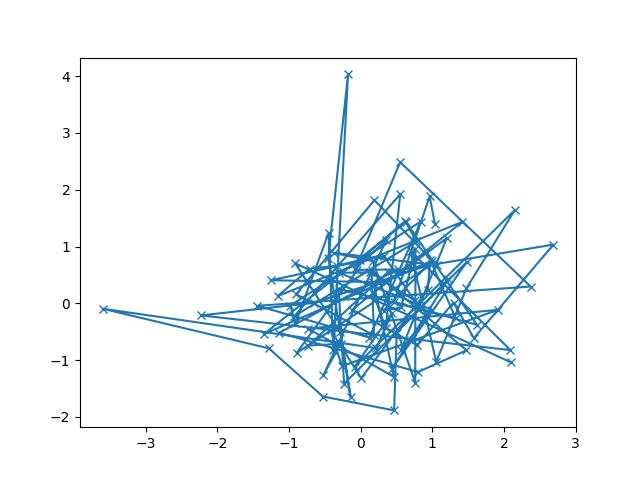Out:

```[<matplotlib.lines.Line2D object at 0x7fd1ed1c05b0>]
```

or if you wanted to have two sub-plots:

```fig, (ax1, ax2) = plt.subplots(1, 2)
my_plotter(ax1, data1, data2, {'marker': 'x'})
my_plotter(ax2, data3, data4, {'marker': 'o'})
```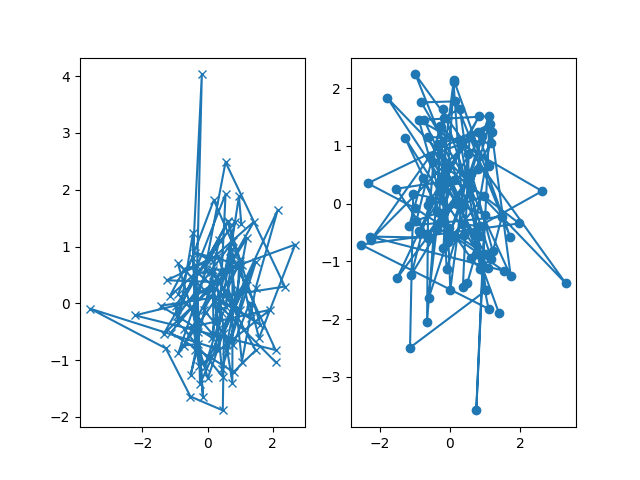Out:

```[<matplotlib.lines.Line2D object at 0x7fd1ed2b3be0>]
```

These examples provide convenience for more complex graphs.

Total running time of the script: ( 0 minutes 3.227 seconds)

Keywords: matplotlib code example, codex, python plot, pyplot Gallery generated by Sphinx-Gallery